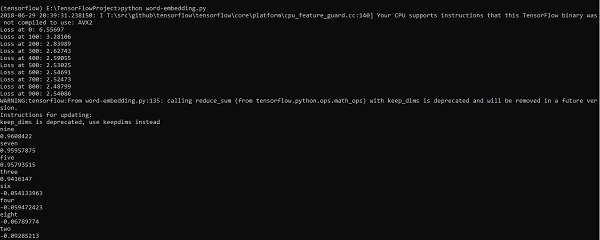# TensorFlow - Word Embedding

Word embedding is the concept of mapping from discrete objects such as words to vectors and real numbers. It is important for input for machine learning. The concept includes standard functions, which effectively transform discrete input objects to useful vectors.

The sample illustration of input of word embedding is as shown below −

blue: (0.01359, 0.00075997, 0.24608, ..., -0.2524, 1.0048, 0.06259)
blues: (0.01396, 0.11887, -0.48963, ..., 0.033483, -0.10007, 0.1158)
orange: (-0.24776, -0.12359, 0.20986, ..., 0.079717, 0.23865, -0.014213)
oranges: (-0.35609, 0.21854, 0.080944, ..., -0.35413, 0.38511, -0.070976)


## Word2vec

Word2vec is the most common approach used for unsupervised word embedding technique. It trains the model in such a way that a given input word predicts the word’s context by using skip-grams.

TensorFlow enables many ways to implement this kind of model with increasing levels of sophistication and optimization and using multithreading concepts and higher-level abstractions.

import os
import math
import numpy as np
import tensorflow as tf

from tensorflow.contrib.tensorboard.plugins import projector
batch_size = 64
embedding_dimension = 5
negative_samples = 8
LOG_DIR = "logs/word2vec_intro"

digit_to_word_map = {
1: "One",
2: "Two",
3: "Three",
4: "Four",
5: "Five",
6: "Six",
7: "Seven",
8: "Eight",
9: "Nine"}
sentences = []

# Create two kinds of sentences - sequences of odd and even digits.
for i in range(10000):
rand_odd_ints = np.random.choice(range(1, 10, 2), 3)
sentences.append(" ".join([digit_to_word_map[r] for r in rand_odd_ints]))
rand_even_ints = np.random.choice(range(2, 10, 2), 3)
sentences.append(" ".join([digit_to_word_map[r] for r in rand_even_ints]))

# Map words to indices
word2index_map = {}
index = 0

for sent in sentences:
for word in sent.lower().split():

if word not in word2index_map:
word2index_map[word] = index
index += 1
index2word_map = {index: word for word, index in word2index_map.items()}

vocabulary_size = len(index2word_map)

# Generate skip-gram pairs
skip_gram_pairs = []

for sent in sentences:
tokenized_sent = sent.lower().split()

for i in range(1, len(tokenized_sent)-1):
word_context_pair = [[word2index_map[tokenized_sent[i-1]],
word2index_map[tokenized_sent[i+1]]], word2index_map[tokenized_sent[i]]]

skip_gram_pairs.append([word_context_pair, word_context_pair])
skip_gram_pairs.append([word_context_pair, word_context_pair])

def get_skipgram_batch(batch_size):
instance_indices = list(range(len(skip_gram_pairs)))
np.random.shuffle(instance_indices)
batch = instance_indices[:batch_size]
x = [skip_gram_pairs[i] for i in batch]
y = [[skip_gram_pairs[i]] for i in batch]
return x, y

# batch example
x_batch, y_batch = get_skipgram_batch(8)
x_batch
y_batch
[index2word_map[word] for word in x_batch] [index2word_map[word] for word in y_batch]

# Input data, labels train_inputs = tf.placeholder(tf.int32, shape = [batch_size])
train_labels = tf.placeholder(tf.int32, shape = [batch_size, 1])

# Embedding lookup table currently only implemented in CPU with
tf.name_scope("embeddings"):
embeddings = tf.Variable(
tf.random_uniform([vocabulary_size, embedding_dimension], -1.0, 1.0),
name = 'embedding')
# This is essentialy a lookup table
embed = tf.nn.embedding_lookup(embeddings, train_inputs)

# Create variables for the NCE loss
nce_weights = tf.Variable(
tf.truncated_normal([vocabulary_size, embedding_dimension], stddev = 1.0 /
math.sqrt(embedding_dimension)))

nce_biases = tf.Variable(tf.zeros([vocabulary_size]))

loss = tf.reduce_mean(
tf.nn.nce_loss(weights = nce_weights, biases = nce_biases, inputs = embed,
labels = train_labels,num_sampled = negative_samples,
num_classes = vocabulary_size)) tf.summary.scalar("NCE_loss", loss)

# Learning rate decay
global_step = tf.Variable(0, trainable = False)
learningRate = tf.train.exponential_decay(learning_rate = 0.1,
global_step = global_step, decay_steps = 1000, decay_rate = 0.95, staircase = True)

merged = tf.summary.merge_all()
with tf.Session() as sess:
train_writer = tf.summary.FileWriter(LOG_DIR,
graph = tf.get_default_graph())
saver = tf.train.Saver()

metadata.write('Name\tClass\n') for k, v in index2word_map.items():

config = projector.ProjectorConfig()
embedding = config.embeddings.add() embedding.tensor_name = embeddings.name

projector.visualize_embeddings(train_writer, config)

tf.global_variables_initializer().run()

for step in range(1000):
x_batch, y_batch = get_skipgram_batch(batch_size) summary, _ = sess.run(
[merged, train_step], feed_dict = {train_inputs: x_batch, train_labels: y_batch})

if step % 100 == 0:
saver.save(sess, os.path.join(LOG_DIR, "w2v_model.ckpt"), step)
loss_value = sess.run(loss, feed_dict = {
train_inputs: x_batch, train_labels: y_batch})
print("Loss at %d: %.5f" % (step, loss_value))

# Normalize embeddings before using
norm = tf.sqrt(tf.reduce_sum(tf.square(embeddings), 1, keep_dims = True))
normalized_embeddings = embeddings /
norm normalized_embeddings_matrix = sess.run(normalized_embeddings)

ref_word = normalized_embeddings_matrix[word2index_map["one"]]

cosine_dists = np.dot(normalized_embeddings_matrix, ref_word)
ff = np.argsort(cosine_dists)[::-1][1:10] for f in ff: print(index2word_map[f])
print(cosine_dists[f])


### Output

The above code generates the following output −